# Catch up on the latest AI articles# Unsupervised Tuning Of Domain Adaptation Models

3 Key Points.
✔️ Unsupervised Domain Adaptation has high prediction performance on the target data, but the lack of supervised data makes it difficult to tune hyperparameters
✔️ In this paper, we devised a novel evaluation metric, Soft Neighborhood Density (SND), under the assumption that target data of the same class are embedded in each other's neighborhood by the discriminator
✔️ SND was found to be more effective in both image recognition and segmentation, despite its simplicity compared to traditional methods

written by Kuniaki SaitoDonghyun KimPiotr TeterwakStan SclaroffTrevor DarrellKate Saenko
(Submitted on 24 Aug 2021)

Subjects: Computer Vision and Pattern Recognition (cs.CV)code：The images used in this article are from the paper, the introductory slides, or were created based on them.

## first of all

While deep neural networks can learn very accurate features in image recognition, they cannot maintain their performance in different domains. To improve this, unsupervised Domain Adaptation (UDA) has been studied; UDA learns features on unsupervised target data from source data with sufficient supervised data. In recent years, various UDA methods have been developed and have shown high performance in image recognition, semantic segmentation and object recognition. However, the performance of UDA varies greatly depending on the hyperparameters and the number of training iterations, which makes evaluation important.Since UDA does not have any target supervisory data, how should we do hyperparameter tuning (HPO)?

A conventional and effective method is Classification Entropy (C-Ent). If the classifier is confident in its output and has low entropy, then the features in the target data should be easy to identify and result in a reliable classification. However, C-Ent will also be low in cases where the classifier is confidently classifying some of the target data into a different class, as shown in the figure below.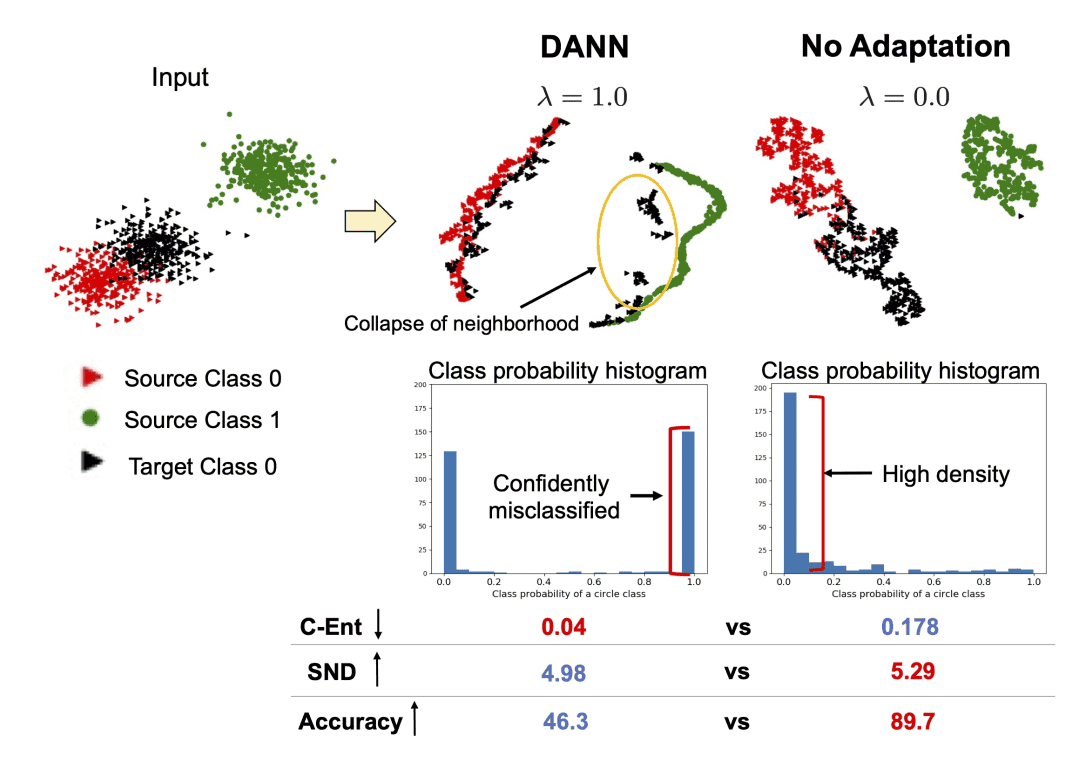In this paper, we define Soft Neighborhood Density (SND) under the assumption that a well-trained model incorporates target data of the same class nearby, forming a dense neighborhood of points. SND is a simple measure of the discriminability of target data features by calculating the density of neighborhoods.

## problem-setting

If the source data is $D_s=\{({\bf x}_i^s, y_i^s)}_{i=1}^{N_s}$ and the target data is $D_t=\{({\bf x}_i^t)}_{i=1}^{N_t}$, the loss of UDA is generally

$$L = L_s(x_s, y_s)+\lambda L_{adapt}(x_s, x_t, \eta)$$.

can be written as where $L_s$ is the classification loss of the source data, $L_{adapt}$ is the adaptation loss of the target data, $\lambda$ is the trade-off parameter, and $\eta$ is the hyperparameter for computing $L_{adapt}$. Our goal is to find an evaluation metric that optimizes $\lambda$, $\eta$, and the number of training iterations.

## Soft Neighborhood Density (SND)

The derivation of the SND consists of three steps: 1) Similarity calculation, 2) Soft Neighborhoods calculation, and 3) SND calculation, as shown in the figure below.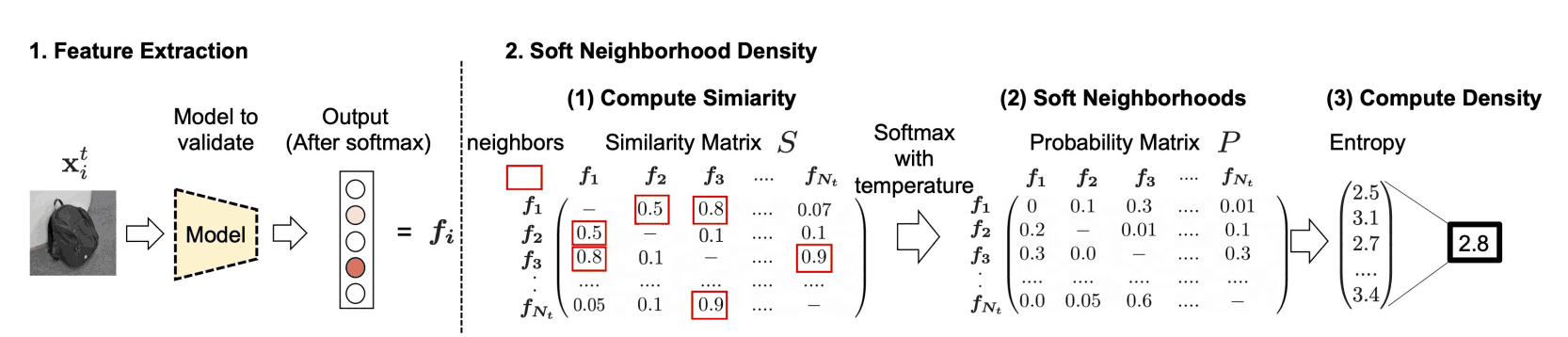### similarity

First, calculate the similarity from the target sample $S\in \mathbb{R}^{N_t\times N_t}$. However, $N_t$ is the number of target samples. Define the similarity matrix S with $S_{ij}=<{\bf f}_i^t, {\bf f}_j^t>$ as the feature value of the target data $\bf{x}_i^t$ with ${\bf f}_i^t$ normed to $L_2$. However, we ignore the diagonal components as they are not necessary. The matrix $S$ defines the distance, but it is unclear how far apart the samples are relative to each other.

### Soft Neighborhoods

Then, to highlight the distances between the samples, we transform them into probability distributions using the Softmax function.

$$P_{ij}=\frac{\exp{(S_{ij}/\tau})}{\sum_{j'}\exp{(S_{ij'}/\tau})}$$.

where $\tau$ is a temperature parameter that adjusts the distance between samples. Thus, if sample $j$ is not relatively similar to sample $i$, $P_{ij}$ will be small and sample $j$ can be ignored. In this experiment, we set $\tau=0.05$.

### SND

Finally, we calculate the entropy of $P$ as a metric to consider neighborhood density when $P$ is given. If the entropy of $P_i$ is large, then the probability distribution should be uniform within soft neighborhoods. That is, the neighborhoods of sample $i$ are densely packed with very close points.

$$H(P)=-\frac{1}{N_t}\sum_{i=1}^{N_t}\sum_{j=1}^{N_t}P_{ij}\log{P_{ij}}$$.

In the case of the distribution shown in the figure at the beginning, $H(P)$ is small, so we selected the model with the largest $H(P)$. Also, since the purpose of SND is to put the same class of the target data in the neighborhood, we used the softmax output of the classifier that contains the class information for ${\bf f}$.

## experimental results

### image classification

Accuracy and evaluation indicators against the number of training iterations are shown in the figure below.

The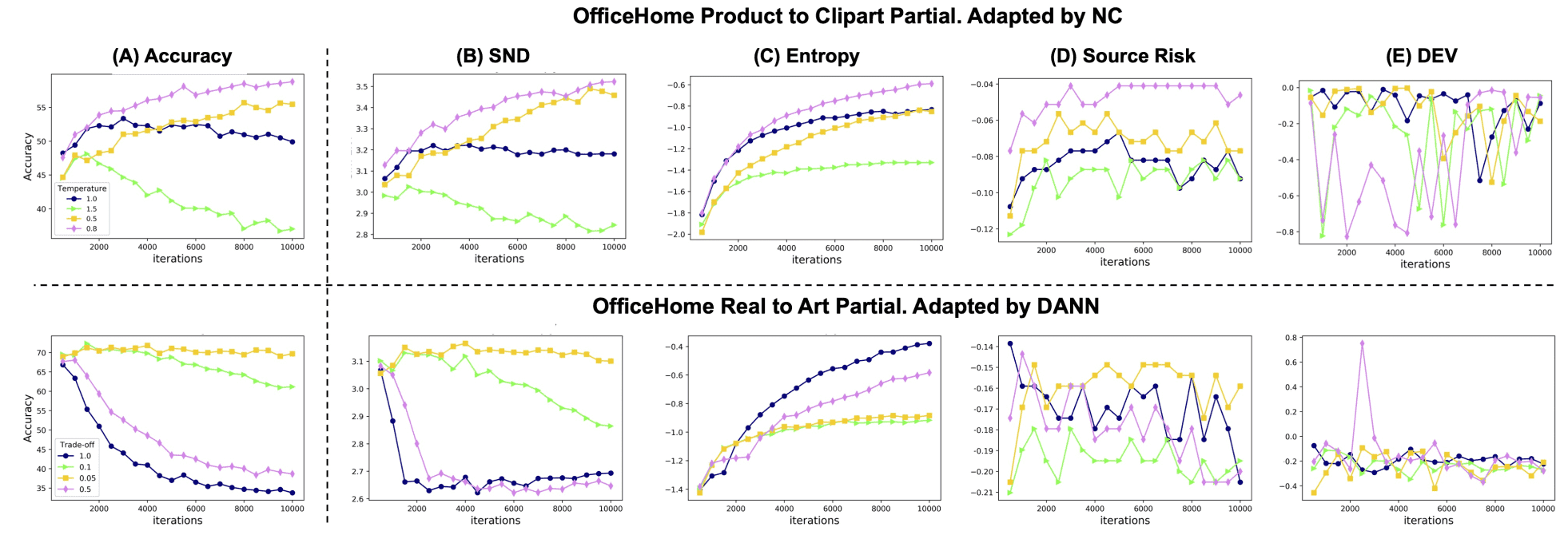Domain Adaptation (DA) method shows a large variation with hyperparameters. However, the curves of SND and Accuracy are similar. The Accuracy results when different DA methods are applied to multiple datasets are shown in the table below.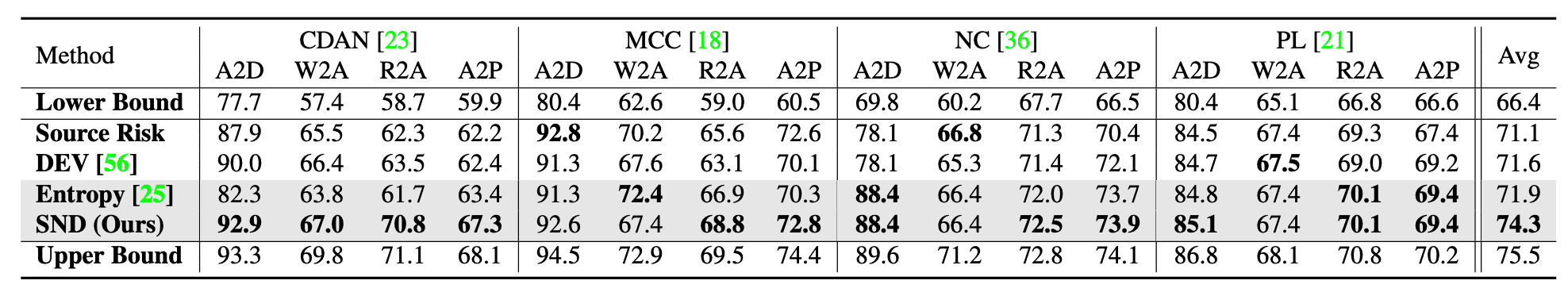CDAN, MCC, NC, and PL represent DA methods, A2D, W2A, R2A, and A2P represent different data sets, and Source Risk, DEV, and Entropy (C-Ent) represent traditional evaluation metrics. Overall, SND has the highest value, and it hardly degrades while other evaluation metrics have significantly lower performance in some cases. All this shows that SND is effective in HPO of various methods.

### semantic segmentation

The experimental results of segmentation are shown in the table below; the DA methods are AdaptSeg and ADVENT, and the datasets are GTA5 and CityScape. The values in the table are mean IoU (mIoU). In both methods, SND has high values.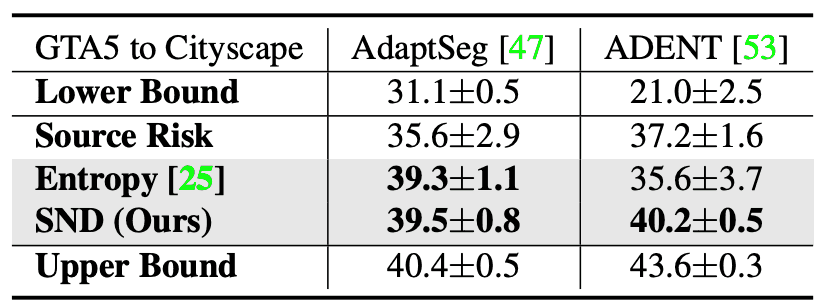## summary

In this paper, we propose SND, which takes into account how well the target samples are clustered, as a better evaluation metric for UDA, and compare it with conventional methods on various datasets. HPO is crucial for UDA methods because their performance varies greatly depending on hyperparameters. Although the SND showed higher performance than the conventional metrics, the unsupervised HPO also varies among DA methods, and establishing a DA method that takes into account how the HPO works is a future challenge.

If you have any suggestions for improvement of the content of the article,